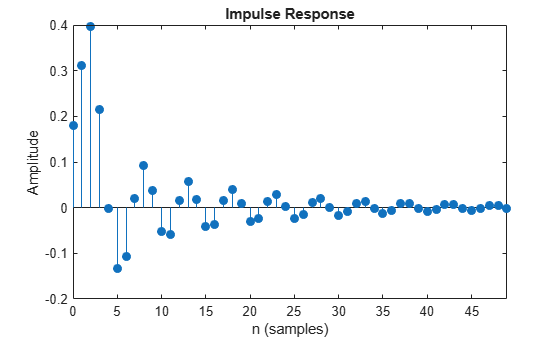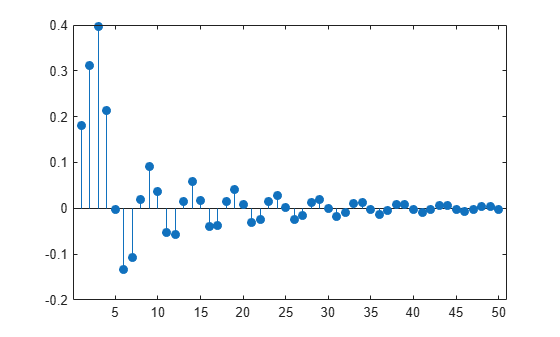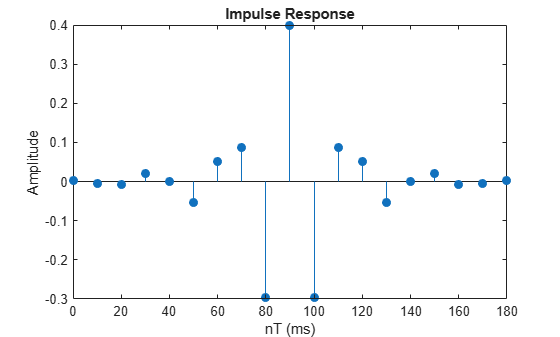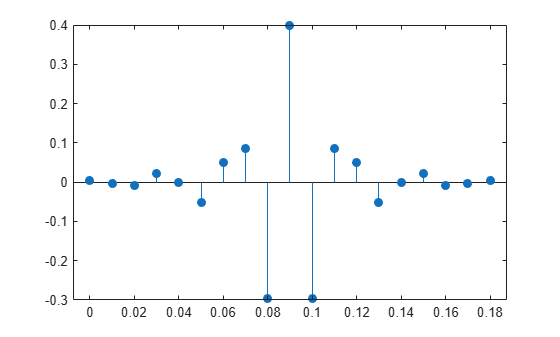# impz

Impulse response of digital filter

## Syntax

``[h,t] = impz(b,a)``
``[h,t] = impz(sos)``
``[h,t] = impz(d)``
``[h,t] = impz(___,n)``
``[h,t] = impz(___,n,fs)``
``impz(___)``

## Description

example

````[h,t] = impz(b,a)` returns the impulse response of the digital filter with numerator coefficients `b` and denominator coefficients `a`. The function chooses the number of samples and returns the response coefficients in `h` and the sample times in `t`.```
````[h,t] = impz(sos)` returns the impulse response of the filter specified by the second-order sections matrix `sos`.```

example

````[h,t] = impz(d)` returns the impulse response of the digital filter `d`. Use `designfilt` to generate `d` based on frequency-response specifications.```

example

````[h,t] = impz(___,n)` specifies what impulse-response samples to compute. You can specify the filter using any of the previous syntaxes.```

example

````[h,t] = impz(___,n,fs)` returns a vector `t` with consecutive samples spaced 1/`fs` units apart.```

example

````impz(___)` with no output arguments plots the impulse response of the filter.```

## Examples

collapse all

Design a fourth-order lowpass elliptic filter with normalized passband frequency 0.4 rad/sample. Specify a passband ripple of 0.5 dB and a stopband attenuation of 20 dB. Plot the first 50 samples of the impulse response.

```[b,a] = ellip(4,0.5,20,0.4); impz(b,a,50)```Design the same filter using `designfilt`. Plot the first 50 samples of its impulse response.

```d = designfilt('lowpassiir','DesignMethod','ellip','FilterOrder',4, ... 'PassbandFrequency',0.4, ... 'PassbandRipple',0.5,'StopbandAttenuation',20); impz(d,50)```Design an FIR highpass filter of order 18 using a Kaiser window with $\beta =4$. Specify a sample rate of 100 Hz and a cutoff frequency of 30 Hz. Display the impulse response of the filter.

```b = fir1(18,30/(100/2),'high',kaiser(19,4)); impz(b,1,[],100)```Design the same filter using `designfilt` and plot its impulse response.

```d = designfilt('highpassfir','FilterOrder',18,'SampleRate',100, ... 'CutoffFrequency',30,'Window',{'kaiser',4}); impz(d,[],100)```## Input Arguments

collapse all

Transfer function coefficients, specified as vectors. Express the transfer function in terms of `b` and `a` as

`$H\left({e}^{j\omega }\right)=\frac{B\left({e}^{j\omega }\right)}{A\left({e}^{j\omega }\right)}=\frac{\text{b(1)}+\text{b(2)}\text{\hspace{0.17em}}{e}^{-j\omega }+\text{b(3)}\text{\hspace{0.17em}}{e}^{-j2\omega }+\cdots +\text{b(M)}\text{\hspace{0.17em}}{e}^{-j\left(M-1\right)\omega }}{\text{a(1)}+\text{a(2)}\text{\hspace{0.17em}}{e}^{-j\omega }+\text{a(3)}\text{\hspace{0.17em}}{e}^{-j2\omega }+\cdots +\text{a(N)}\text{\hspace{0.17em}}{e}^{-j\left(N-1\right)\omega }}.$`

Example: `b = [1 3 3 1]/6` and `a = [3 0 1 0]/3` specify a third-order Butterworth filter with normalized 3 dB frequency 0.5π rad/sample.

Data Types: `double` | `single`
Complex Number Support: Yes

Second-order section coefficients, specified as a matrix. `sos` is a K-by-6 matrix, where the number of sections, K, must be greater than or equal to 2. If the number of sections is less than 2, the function treats the input as a numerator vector. Each row of `sos` corresponds to the coefficients of a second-order (biquad) filter. The ith row of `sos` corresponds to `[bi(1) bi(2) bi(3) ai(1) ai(2) ai(3)]`.

Example: `s = [2 4 2 6 0 2;3 3 0 6 0 0]` specifies a third-order Butterworth filter with normalized 3 dB frequency 0.5π rad/sample.

Data Types: `double` | `single`
Complex Number Support: Yes

Digital filter, specified as a `digitalFilter` object. Use `designfilt` to generate a digital filter based on frequency-response specifications.

Example: ```d = designfilt('lowpassiir','FilterOrder',3,'HalfPowerFrequency',0.5)``` specifies a third-order Butterworth filter with normalized 3 dB frequency 0.5π rad/sample.

Sample numbers, specified as a positive integer, a vector of nonnegative integers, or an empty vector.

• If `n` is a positive integer, `impz` computes the first `n` samples of the impulse response and returns `t` as `(0:n-1)'`.

• If `n` is a vector of nonnegative integers, `impz` computes the impulse response at the locations specified in the vector.

• If `n` is an empty vector, `impz` computes the number of samples automatically. See Algorithms for more information.

Example: `impz([2 4 2 6 0 2;3 3 0 6 0 0],5)` computes the first five samples of the impulse response of a Butterworth filter.

Example: `impz([2 4 2 6 0 2;3 3 0 6 0 0],[0 3 2 1 4 5])` computes the first six samples of the impulse response of a Butterworth filter.

Example: `impz([2 4 2 6 0 2;3 3 0 6 0 0],[],5e3)` computes the impulse response of a Butterworth filter designed to filter signals sampled at 5 kHz.

Sample rate, specified as a positive scalar. When the unit of time is seconds, `fs` is expressed in hertz.

Data Types: `double`

## Output Arguments

collapse all

Impulse response coefficients, returned as a column vector.

Sample times, returned as a column vector.

## Algorithms

`impz` filters a length-`n` impulse sequence using

```filter(b,a,[1 zeros(1,n-1)]) ```

and plots the result using `stem`.

Note

If the input to `impz` is single precision, the function computes the impulse response using single-precision arithmetic and returns single-precision output.

When `impz` calculates `n` automatically, the algorithm depends on the properties of the filter:

• FIR filters — `n` is the length of `b`.

• IIR filters — `impz` first finds the poles of the transfer function using `roots`.

• If the filter is unstable, `n` is chosen to be the point at which the term from the largest pole reaches 106 times its original value.

• If the filter is stable, `n` is chosen as the point at which the term from the largest-amplitude pole is 5 × 10–5 times its original amplitude.

• If the filter is oscillatory with poles on the unit circle only, `impz` computes five periods of the slowest oscillation.

• If the filter has both oscillatory and damped terms, `n` is the greater of five periods of the slowest oscillation, or the point at which the term due to the largest pole is 5 × 10–5 times its original amplitude.

`impz` also allows for delays in the numerator polynomial. The number of delays is incorporated into the computation of the number of samples.

## Extended Capabilities

Introduced before R2006a